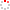CIOMP OpenIR  > 中科院长春光机所知识产出
 Optimization Design and Test for Large Aspect Ratio Rectangular SiC Space Mirror Wei, L.; L. Zhang; Z.-X. Li and D.-M. Ma 2017 Source Publication Guangzi Xuebao/Acta Photonica SinicaVolume 46Issue:3 Abstract According to the characteristics of too large root mean-square value acceleration response of random vibration at edges of rectangular space mirror with large aspect ratio, this paper propose a topological optimization based on ground structure method to design the structure of rectangular space mirror, with the goal for minimizing the acceleration root-mean-square values of random vibration. Firstly, after the finite element method analysis to the initial rectangular space mirror structure, the author find that the acceleration root-mean-square values of random vibration at the edge of mirror is too large to meet the requirements of design. Based on continuum structural topology optimization thought, by using the acceleration root-mean-square values of random vibration at rectangular space mirror's edge as an optimization object, the optical aberration PV and the primary frequency of mirror as the design restraint, the rib plate type ground structure of mirror as the design variable, a topology optimization function is established. Futhermore, the new mirror based on the structure obtained by topology optimization was designed. Finally, by the finite element analysis and vibration test, the new structure of mirror is proved with a good dynamic performance and lightweight rate. Especially, mirror mass has reduced 13%, and the acceleration root-mean-square values of random vibration at mirror's edge in Z direction reduced by 58%. The results demonstrate the validity of proposed approach properly. 2017, Science Press. All right reserved. Indexed By ei Language 中文 Document Type 期刊论文 Identifier http://ir.ciomp.ac.cn/handle/181722/59309 Collection 中科院长春光机所知识产出 Recommended CitationGB/T 7714 Wei, L.,L. Zhang,Z.-X. Li and D.-M. Ma. Optimization Design and Test for Large Aspect Ratio Rectangular SiC Space Mirror[J]. Guangzi Xuebao/Acta Photonica Sinica,2017,46(3). APA Wei, L.,L. Zhang,&Z.-X. Li and D.-M. Ma.(2017).Optimization Design and Test for Large Aspect Ratio Rectangular SiC Space Mirror.Guangzi Xuebao/Acta Photonica Sinica,46(3). MLA Wei, L.,et al."Optimization Design and Test for Large Aspect Ratio Rectangular SiC Space Mirror".Guangzi Xuebao/Acta Photonica Sinica 46.3(2017).